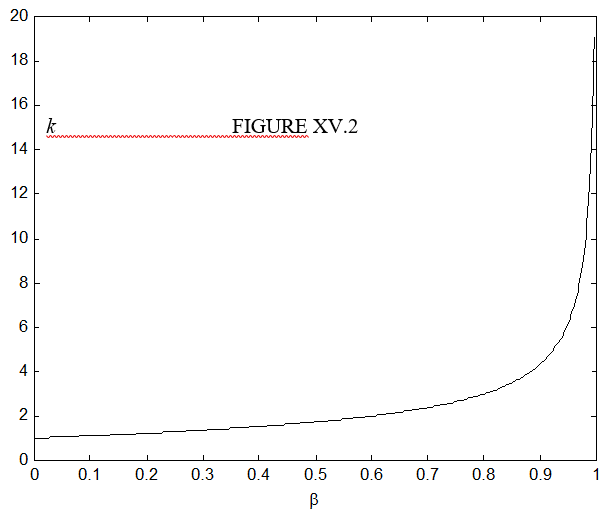$$\require{cancel}$$

# 15.3: Preparation

•• Contributed by Jeremy Tatum
• Emeritus Professor (Physics & Astronomy) at University of Victoria

The ratio of the speed $$\nu$$ of a body (or a particle, or a reference frame) is often given the symbol $$\beta$$:

$\beta=\frac{\nu}{c}. \label{15.3.1}$

For reasons that will become apparent (I hope!) later, the range of $$\beta$$ is usually restricted to between 0 and 1. In our study of special relativity, we shall find that we have to make frequent use of a number of functions of $$\beta$$. The most common of these are

$\gamma=(1-\beta^{2})^{-\frac{1}{2}}, \label{15.3.2}$

$k=\sqrt{\frac{(1+\beta)}{(1-\beta)}}, \label{15.3.3}$

$z=k-1, \label{15.3.4}$

$\phi=\frac{1}{2}\ln \left[\frac{(1+\beta)}{(1-\beta)} \right]=\tanh^{-1}\beta=\ln k. \label{15.3.6}$

$\theta=\cos^{-1}\gamma=\sin^{-1}(i\beta\gamma). \label{15.3.7}$

In Figures XV.1-3 I draw $$\gamma, k$$ and $$\phi$$ as functions of $$\beta$$. The functions $$\gamma$$ and $$k$$ go from 1 to ∞ as b goes from 0 to 1; $$z,K$$ and $$\phi$$ go from 0 to ∞. The function $$\theta$$ is imaginary.

Redundancy

Many – one might even say most – problems in special relativity (including examination and homework questions!) amount, when stripped of their verbiage, to the following:

“Given one of the quantities $$\beta,\gamma,k,z,K,\phi,\theta$$, calculate one of the others.”

Thus I would suggest that, even before you have any idea what these quantities mean, you might write a program for your computer (or programmable calculator) such that, when you enter any one of the real quantities, the computer will instantly return all six of them. This will save you, on future occasions, from having to remember the exact formulas or having to bother with tedious arithmetic, so that you can concentrate your mind on understanding the relativity.Just for future reference, I tabulate here the relations between these various quantities. This has involved some algebra and typesetting; I do not think there are any mistakes, but I hope some reader might check through them all carefully and will let me know (uvic.ca) if he or she finds any.

$$\beta = \sqrt{1-\frac{1}{\gamma^{2}}}=\frac{k^{2}-1}{k^{2}+1}=\frac{z(z+2)}{(z+1)^{2}+1}=\frac{\sqrt{K(K+2)}}{K+1}=\tanh\phi$$ or $$\frac{e^{2\phi}-1}{e^{2\phi}+1}=-i\tan\theta$$

$$\gamma=\frac{1}{\sqrt{1-\beta^{2}}}=\frac{k^{2}+1}{2k}=\frac{(z+1)^{2}+1}{2(z+1)}=K+1=\cosh\phi$$ or $$\frac{1}{2}(e^{\phi}+e^{-\phi})=\cos\theta$$

$$k=\sqrt{\frac{1+\beta}{1-\beta}}=\gamma+\sqrt{\gamma^{2}-1}=z+1=K+1+\sqrt{K(K+2)}=e^{\phi}=e^{-i\theta}$$

$$z=\sqrt{\frac{1+\beta}{1-\beta}}-1=\gamma-1+\sqrt{\gamma^{2}-1}=k-1=K+\sqrt{K(K+2)}=e^{\phi}-1=e^{-i\theta}-1$$

$$K=\frac{1}{\sqrt{1-\beta^{2}}}-1=\gamma-1=\frac{(k-1)^{2}}{2k}=\frac{z^{2}}{2(z+1)}=\frac{(e^{\phi}-1)^{2}}{2e^{\phi}}=\cos\theta-1$$

$$\phi=\tanh^{-1}\beta$$ or $$\frac{1}{2}\ln\left(\frac{1+\beta}{1-\beta}\right)=\cosh^{-1}\gamma$$ or$$\ln(\gamma+\sqrt{\gamma^{2}-1})=\ln k=\ln(z+1)=\ln\left(K+1+\sqrt{K(K+2)}\right)=-i\theta$$

$$\theta=\frac{i}{2}\ln\left(\frac{1+\beta}{1-\beta}\right)=i\ln(\gamma+\sqrt{\gamma^{2}-1})=i\ln k=i\ln(z+1)=i\ln\left[K+1+\sqrt{K(K+2)}\right]=i\phi$$